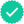Question

The rectangles in the graph below illustrate a left endpoint Riemann sum for f(x)=(x^2)/(12)     f(x)=(x^2)/(12) on the interval [2,6].
The value of this left endpoint Riemann sum is __________________ , the area of the region enclosed by y=f(x)y=f(x), the x-axis, and the vertical lines x = 2 and x = 6.

Expert Solution